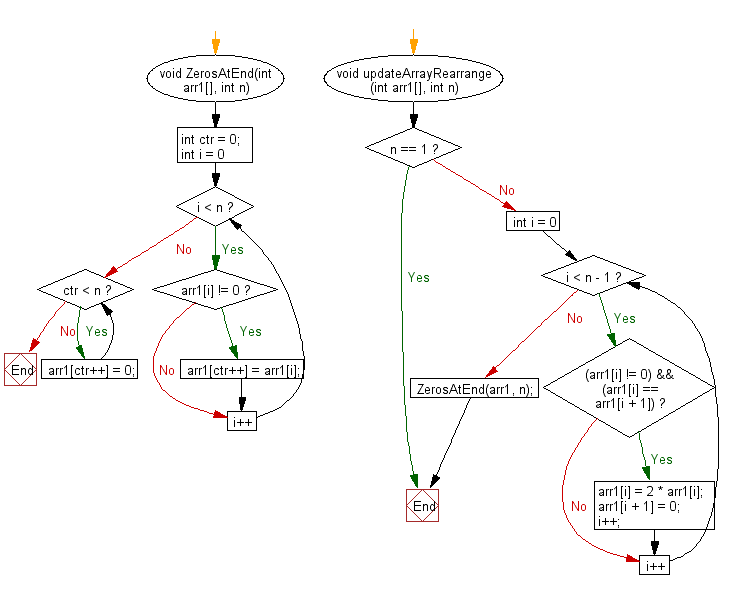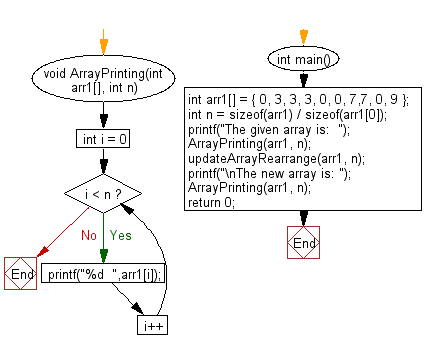﻿ C exercises: Double its value and replace the next number with 0 if current and next value are same and shift all 0's to the end - w3resource# C Exercises: Double its value and replace the next number with 0 if current and next value are same and shift all 0's to the end

## C Array: Exercise-106 with Solution

Write a program in C to convert the array in such a way that double its value and replace the next number with 0 if current and next element are same and rearrange the array such that all 0's shifted to the end.

Sample Solution:

C Code:

``````#include<stdio.h>
void ZerosAtEnd(int arr1[], int n)
{
int ctr = 0;
for (int i = 0; i < n; i++)
if (arr1[i] != 0)
arr1[ctr++] = arr1[i];
while (ctr < n)
arr1[ctr++] = 0;
}
void updateArrayRearrange(int arr1[], int n)
{
if (n == 1)
return;
for (int i = 0; i < n - 1; i++)
{
if ((arr1[i] != 0) && (arr1[i] == arr1[i + 1]))
{
arr1[i] = 2 * arr1[i];
arr1[i + 1] = 0;
i++;
}
}
ZerosAtEnd(arr1, n);
}
void ArrayPrinting(int arr1[], int n)
{
for (int i = 0; i < n; i++)
printf("%d  ",arr1[i]);
}

int main()
{
int arr1[] = { 0, 3, 3, 3, 0, 0, 7,7, 0, 9 };
int n = sizeof(arr1) / sizeof(arr1);
printf("The given array is:  ");
ArrayPrinting(arr1, n);
updateArrayRearrange(arr1, n);
printf("\nThe new array is: ");
ArrayPrinting(arr1, n);
return 0;
}
```
```

Sample Output:

```The given array is:  0  3  3  3  0  0  7  7  0  9
The new array is: 6  3  14  9  0  0  0  0  0  0
```

Flowchart 1:Flowchart 2:C Programming Code Editor:

Improve this sample solution and post your code through Disqus.

﻿

## C Programming: Tips of the Day

Where is the C auto keyword used?

auto is a modifier like static. It defines the storage class of a variable. However, since the default for local variables is auto, you don't normally need to manually specify it.

Ref : https://bit.ly/3yzwC9r